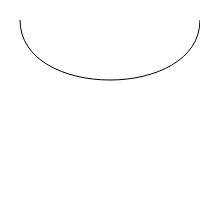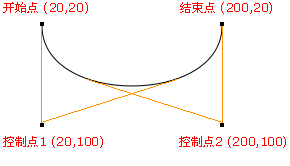# HTML5 Canvas : Bezier Curve Of Path

## What is Bezier curve?

Bezier curve is such a curve. It is a smooth curve drawn according to the coordinates of any point in four positions. In history, the people who studied Bezier curve first designed this vector curve drawing method according to the idea of determining four points according to the known curve parameter equation.

What's more interesting about Bezier curve is its "tendon effect", that is to say, with the regular movement of points, the curve will produce the same transformation of tendon extension, bringing visual impact.

In 1962, Pierre B é zier, a French mathematician, first studied the method of drawing curve by vector and gave detailed calculation formula. Therefore, the curve drawn by this formula was named Bessel curve by his surname.

## Path diagram

Linear Bezier curve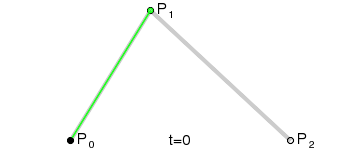cubic Bezier curve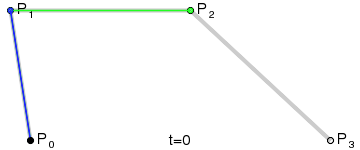A quadratic Bezier curve requires two points. The first point is the control point used in the quadratic Bessel calculation, and the second point is the end point of the curve. The start point of the curve is the last point in the current path. If the path does not exist, you need to use the `beginpath()` and `moveto()` methods to define the start point.

``````//Create quadratic Bezier curve
//cpx: X coordinate of Bezier control point
//cpy: Y coordinate of Bezier control point
//x: X coordinate of the end point
//y: Y coordinate of the end point
``````
``````var canvas=document.getElementById("myCanvas");
var ctx=canvas.getContext("2d");
ctx.beginPath();
ctx.moveTo(20,20);
ctx.stroke();
``````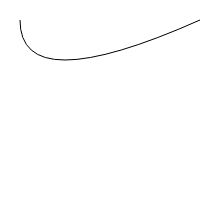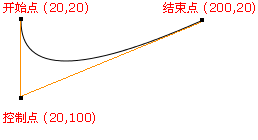## Draw cubic Bezier curve

A cubic Bezier curve requires three points. The first two points are the control points used in the cubic Bezier calculation, and the third point is the end point of the curve. The start point of the curve is the last point in the current path. If the path does not exist, you need to use the beginpath() and moveto() methods to define the start point.

``````//Create cubic Bezier curve
//cp1x: X coordinate of the first Bezier control point
//cp1y: Y coordinate of the first Bezier control point
//cp2x: X coordinate of the second Bezier control point
//cp2y: Y coordinate of the second Bezier control point
//x: X coordinate of the end point
//y: Y coordinate of the end point
context.bezierCurveTo(cp1x, cp1y, cp2x, cp2y, x, y)
``````
``````var canvas=document.getElementById("myCanvas");
var ctx=canvas.getContext("2d");
ctx.beginPath();
ctx.moveTo(20,20);
ctx.bezierCurveTo(20,100,200,100,200,20);
ctx.stroke();
``````